As an Amazon Associate I earn from qualifying purchases.

# What is a Demultiplexer (Demux)?

In this tutorial, we will learn about an interesting Digital Logic Circuit called the Demultiplexer, also known as Demux. Learn what is a Demultiplexer, what are the different types of commonly used Demux configurations like 1-to-2, 1-to-4, 1-to-8 and also some applications of Demultiplexer.

## Introduction

In the previous tutorial, we learned about Multiplexers or simply MUX. Multiplexers are simple combinational logic circuit, which selects one of many inputs and passes it through the single output. MUX is the device responsible for Multiplexing.

The action or operation of a Demultiplexer is exactly the opposite to that of a Multiplexer. As an inverse to the MUX, Demux is a one-to-many circuit. With the use of a Demultiplexer, data from one input can be passed to one of the many output data lines.

Demultiplexers are mainly used in Boolean function generators and decoder circuits. Different input/output configuration demultiplexers are available in the form of single integrated circuits (ICs).

Also, there is a facility of cascading two or more DEMUX circuits, to generate multiple output demultiplexers. Let us get a brief idea of demultiplexers and its types in this tutorial.

## What is a Demultiplexer?

The process of getting information from one input and transmitting the same over one of many outputs is called Demultiplexing. If you recall the Multiplexer tutorial, there we discussed the concept of Multiplexing. Demultiplexing is just the opposite of that.

A Demultiplexer is a combinational logic circuit that receives the information on a single input line and transmits the same information over one of ‘n’ possible output lines.

In order to select a particular output, we have to use a set of Select Lines and the bit combinations of these select lines control the selection of specific output line to be connected to the input at a given instant. The below figure illustrates the basic idea of demultiplexer, in which the switching of the input to any one of the four outputs is possible at a given instant.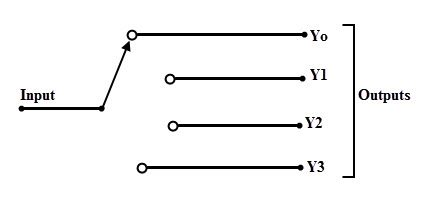If Multiplexers are called as Data Selectors, then Demultiplexers are called as Data Distributors, since they transmit the same data which is received at the input to different destinations.

Thus, a demultiplexer is a 1-to-N device, where as the multiplexer is an N-to-1 device. The figure below shows the block diagram of a demultiplexer or simply a DEMUX.

It consists of 1 input line, ‘n’ output lines and ‘m’ select lines. In this, m select lines are required to produce 2m possible output lines (consider 2m = n). For example, a 1-to-4 demultiplexer requires 2 (22 = 4) select lines to control the 4 output lines.There are several types of demultiplexers based on the output configurations such as 1:2, 1:4, 1:8 and 1:16.

These are available in different IC packages and some of the most commonly used demultiplexer ICs includes 74139 (dual 1:4 DEMUX), 74138 (1:8 DEMUX), 74237 (1:8 DEMUX with Address Latches), 74154 (1:16 DEMUX), 74159 (1:16 DEMUX open collector type), etc.

NOTE: The Demultiplexer ICs are also called as Decoder ICs. For example, 74159 is a 4-line to 16-line Decoder IC.

## 1-to-2 Demultiplexer

A 1-to-2 demultiplexer consists of one input line, two output lines and one select line. The signal on the select line helps to switch the input to one of the two outputs. The figure below shows the block diagram of a 1-to-2 demultiplexer with additional enable input.

In the figure, there are only two possible ways to connect the input to output lines, thus only one select signal is enough to do the demultiplexing operation. When the select input is LOW, then the input will be passed to Y0 and if the select input is HIGH, then the input will be passed to Y1.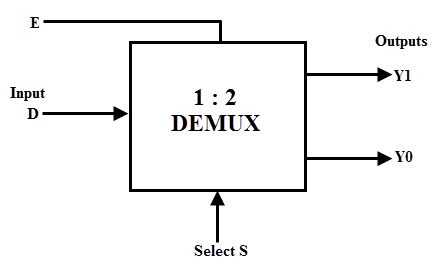The truth table of a 1-to-2 demultiplexer is shown below, in which the input is routed to Y0 and Y1 depending on the value of select input S.

We can derive the Boolean Expressions for the outputs as follows:

Assume S is the Select Input, D is the Data Input and Y0 and Y1 are the outputs of the 1-to-2 Demultiplexer. From the above table, the output Y0 is active when the combination of select line and input line are active low and high respectively, i.e.,  S D = 0 1 .

Therefore, the expression for output Y0 is

Y0 = S

Similarly, the output Y1 is active when the combination of select line and input line are active high, i.e.,  S D = 1 1 .

Therefore, the expression for output Y0 is

Y1 = S D

From the above truth table and the derived Boolean Expressions, the logic diagram of 1-to-2 demultiplexer can be designed using two AND gates and one NOT gate as shown in below figure. When the select line S = 0, the first AND gate (A1) is enabled, while the second AND gate (A2) is disabled.

Then, the data from the input flows to the output line Y0. Similarly, when S = 1, the second AND gate (A2) is enabled and the first AND gate (A1) is disabled, thus data is passed to the Y1 output.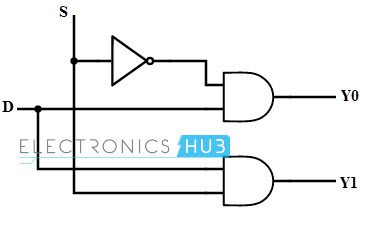## 1-to-4 Demultiplexer

A 1-to-4 demultiplexer has a single input (D), two selection lines (S1 and S0) and four outputs (Y0 to Y3). The input data goes to any one of the four outputs at a given time for a particular combination of select lines.

This demultiplexer is also called as a 2-to-4 Demultiplexer, which means that it has two select lines and 4 output lines. The block diagram of a 1:4 DEMUX is shown below.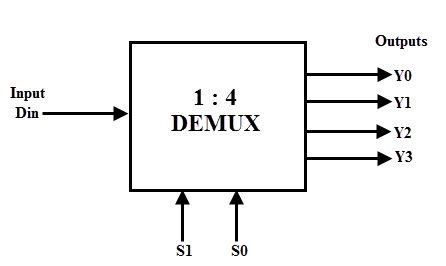The truth table of this type of demultiplexer is given below. From the truth table it is clear that, when S0 = 0 and S1 = 0, the data input is connected to output Y0 and when S0 = 0 and s1=1, the data input is connected to output Y1.

Similarly, other outputs are connected to the input for the other two combinations of select lines.

 S1 S0 D Y3 Y2 Y1 Y0 0 0 0 0 0 0 0 0 0 1 0 0 0 1 0 1 0 0 0 0 0 0 1 1 0 0 1 0 1 0 0 0 0 0 0 1 0 1 0 1 0 0 1 1 0 0 0 0 0 1 1 1 1 0 0 0

From the above truth table, we can derive the Boolean Expressions for the outputs as follows:

Y0 = S1 S0

Y1 = S1 S0 D

Y2 = S1 S0

Y3 = S1 S0 D

Where D is the input data, Y0 to Y3 are output lines and S0 & S1 are select lines.

From the above Boolean expressions, a 1-to-4 demultiplexer can be implemented by using four 3-input AND gates and two NOT gates as shown in figure below. The two select lines enable a particular AND gate at a time. Additionally, there is an Enable / Strobe Input, which acts as a global enable input i.e., the outputs are active only when the ‘E’ bit is HIGH.

So depending on the combination of select inputs, input data is passed through the selected gate to the associated output.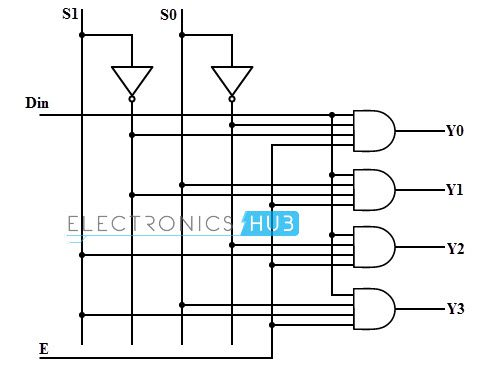This type of demultiplexer is available in integrated circuit form as IC 74139. It one of the most commonly used demultiplexer ICs and it is a dual 1-to-4 demultiplexer IC i.e., it contains two independent 1-to-4 demultiplexer blocks in one IC.  Each DEMUX accepts two binary inputs as select lines and four mutually exclusive active-low outputs.

Both demultiplexers have individual set of select lines so they can acts as truly independent Demux. Also, each demultiplexer consists of dedicated enable pin, which can act as a data input for the demultiplexer operation. The Enable pins are active LOW.The outputs are active LOW i.e., they are HIGH by default. So, if the Enable pin is HIGH, all the outputs are HIGH and if Enable is LOW, then based on the Select Pins, only the corresponding output pin becomes LOW.

## 1-to-8 Demultiplexer

The below figure shows the block diagram of a 1-to-8 demultiplexer that consists of single input D, three select inputs S2, S1 and S0 and eight outputs from Y0 to Y7.

It is also called as 3-to-8 demultiplexer due to its three select input lines and 8 output lines. It distributes one input line to one of 8 output lines depending on the combination of select inputs.The truth table for 1-to-8 demultiplexer is shown below. The input ‘D’ is connected with one of the eight outputs from Y0 to Y7 based on the select lines S2, S1 and S0.

For example, if S2 S1 S0 = 0 0 0, then the input D is connected to the output Y0 and so on.

 S2 S1 S0 Y7 Y6 Y5 Y4 Y3 Y2 Y1 Y0 0 0 0 0 0 0 0 0 0 0 D 0 0 1 0 0 0 0 0 0 D 0 0 1 0 0 0 0 0 0 D 0 0 0 1 1 0 0 0 0 D 0 0 0 1 0 0 0 0 0 D 0 0 0 0 1 0 1 0 0 D 0 0 0 0 0 1 1 0 0 D 0 0 0 0 0 0 1 1 1 D 0 0 0 0 0 0 0

From this truth table, the Boolean expressions for all the outputs can be written as follows.

Y0 = S2 S1 S0

Y1 = S2 S1 S0 D

Y2 = S2 S1 S0

Y3 = S2 S1 S0 D

Y4 = S2 S1 S0

Y5 = S2 S1 S0 D

Y6 = S2 S1 S0

Y7 = S2 S1 S0 D

From these obtained equations, the logic diagram of this demultiplexer can be implemented by using eight 4-input AND gates and three NOT gates as shown in below figure. Different combinations of the select lines activates one AND gate at given time, such that data input will appear at the corresponding output.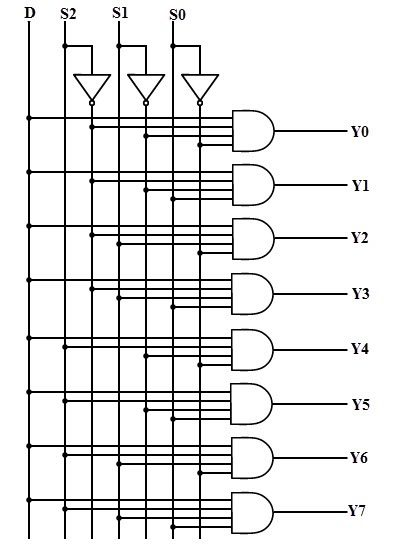There are two popular 1-to-8 demultiplexer integrated circuits. One is the IC 74237, that consists of latches at three select inputs. The pin out of this IC is given below.

The pins A0 to A2 are data inputs, Y0 to Y7 are demultiplexer outputs, E1&E2 are active-low data enable and active-high data enable pins respectively, LE is the latch enable input ,Vcc and GND terminals are positive supply voltage and ground terminals.

This IC combines a 3-bit storage latch with the 3-to-8 decoder function.Another commonly used 1-to-8 demultiplexer integrated circuits is the IC 74138. The pinout is very similar except that there is no Latch Enable input (as all the enable pins are normal enable pins – two are active LOW and one is active HIGH) and the outputs are active LOW. The following image shows the pinout of 74138 IC.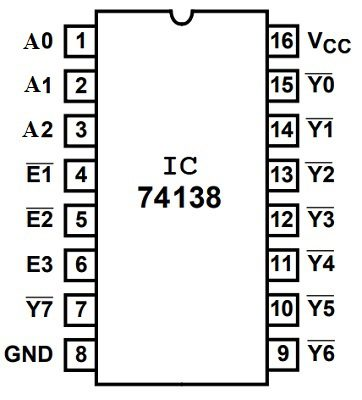### 1-to-8 DEMUX using Two 1-to- 4 Demultiplexers

When the application requires a higher order demultiplexer with more number of output pins, then we cannot implement by a single integrated circuit. In case if more than 16 output pins are needed, then two or more demultiplexer ICs are cascaded to fulfill the requirement.

For example, if the application needs 32 output lines from a DEMUX, then we cascade two 1:16 demultiplexers or three 1:8 demultiplexers. Therefore, by cascading the two or more demultiplexers, a large demultiplexer can be implemented.

Consider the case that a 1-to-8 demultiplexer can be implemented by using two 1-to-4 demultiplexers with a proper cascading.In the above figure, the highest significant bit A of the selection inputs are connected to the enable inputs such that it is complemented before connecting to one DEMUX and to the other it is directly connected.

By this configuration, when A is set to zero, one of the output lines from Y0 to Y3 is selected based on the combination of select lines B and C. Similarly, when A is set to one, based on the select lines one of the output lines from Y4 to Y7 will be selected.

### Implementation of Full Subtractor Using 1-to-8 DEMUX

Similar to the multiplexers, demultiplexers are also used for Boolean function implementation as well as combinational circuit design. We can design a demultiplexer to produce any truth table output by properly controlling the select lines.

Consider the case for implementing a demultiplexer circuit in order to produce the full subtractor output. The truth table below shows the output of a full subtractor.

 A B BIN D BOUT 0 0 0 0 0 0 0 1 1 1 0 1 0 1 1 0 1 1 0 1 1 0 0 1 0 1 0 1 0 0 1 1 0 0 0 1 1 1 1 1

From the above table, the full subtractor output D can be written as

D = f (A, B, BIN

D = ∑m (1, 2, 4, 7)

D = A B BIN + A B BIN + A B BIN + A B BIN

And the borrow output can be expressed as

BOUT = f (A, B, BIN) = ∑m (1, 2, 3, 7)

BOUT = A B BIN + A B BIN + A B BIN + A B BIN

From these Boolean expressions, a demultiplexer for producing full subtractor output can be built by properly configuring the 1-to-8 DEMUX, such that with input D = 1, it gives the minterms at the output.

And by logically ORing these minterms, the outputs of difference and borrow can be obtained as shown in figure.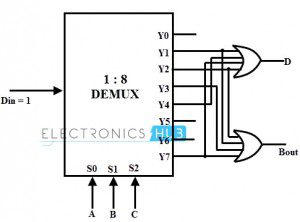## Applications of Demultiplexer

Since the demultiplexers are used to select or enable the one signal out of many, these are extensively used in microprocessor or computer control systems such as:

• Selecting different IO devices for data transfer (Data Routing)
• Choosing different banks of memory (Memory Decoding)
• Depends on the address, enabling different rows of memory chips
• Enabling different functional units.

Other than these, demultiplexers can be found in a wide variety of application such as:

• Synchronous data transmission systems
• Boolean function implementation (as we discussed full subtractor function above)
• Data acquisition systems
• Combinational circuit design
• Automatic test equipment systems
• Security monitoring systems (for selecting a particular surveillance camera at a time), etc.

## Conclusion

A complete beginner’s guide on Demultiplexers or DEMUX. You learned what is a Demultiplexer, different types of Demux like 1-to-2, 1-to-4, 1-to-8, 1-to-16, their logic circuits and some important applications of Demultiplexers.

We will be happy to hear your thoughts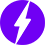Enable registration in settings - general
• Total (0)
0